# YEAR Function

Returns a four-digit year number from a given date

## What is the YEAR Function?

The YEAR function is an Excel Date/Time function that is used for calculating the year number from a given date. The function will return an integer that is a four-digit year corresponding to a specified date. For example, if we use this function on a date such as 12/12/2017, it will return 2017.

Using dates in financial modeling is very common and the YEAR function helps extract a year number from a date into a cell. The function can also be used to extract and feed a year value into another formula such as the DATE function.

### Formula

=YEAR(serial_number)

The serial_number argument is required. It is the date of the year that we wish to find. Dates should be entered either by using the DATE function or as results of other formulas or functions. Problems can occur if dates are entered as text.

### How to use the YEAR Function in Excel?

It is a built-in function that can be used as a worksheet function in Excel. To understand the uses of the YEAR function, let’s consider a few examples:

#### Example 1

Let’s assume we shared an Excel file with several users. We want to ensure that a user enters only dates in a certain year. In such a scenario, we can use data validation with a custom formula based on the YEAR function.

Suppose we are given the following data: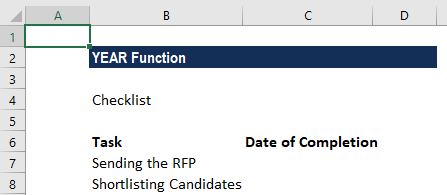Using the data below, we want that user needs to enter only dates in B4 and B5. For this, we will set a data validation rule as follows: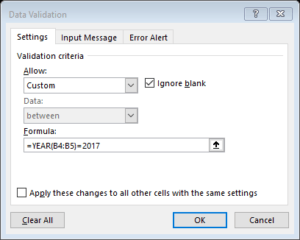Data validation rules will be triggered when any user tries to add or change the cell value.

When the years match, the expression returns TRUE and the validation succeeds. If the years don’t match, or if the YEAR function is not able to extract a year, the validation will fail.

The custom validation formula will simply check the year of any date against a hard-coded year value using the YEAR function and return an error if the year is not entered. If we want to make sure that a user enters only a date in the current year then the formula to be used is =YEAR(C5)=YEAR(TODAY()).

The TODAY function will return the current date on an ongoing basis, so the formula returns TRUE only when a date is in the current year.

#### Example 2

If we want, we can extract the year from a given set of dates. Suppose we wish to extract Year from the dates below: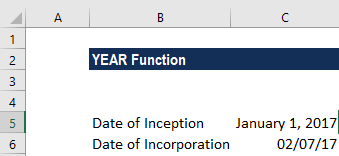Using the YEAR formula, we can extract the year. The formula to use is: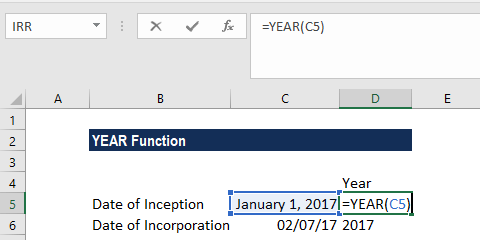We will get the result below: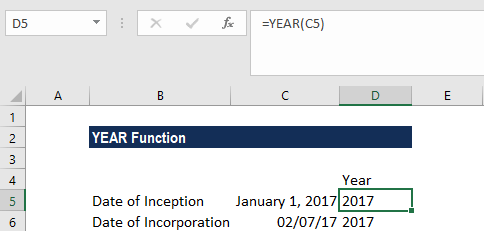### Things to remember about the YEAR Function

1. Dates should be supplied to Excel functions as either:
• Serial numbers; or
• Reference to cells containing dates; or
• Date values returned from other Excel formulas.

If we try to input dates as text, then there is a risk that Excel may misinterpret them, due to the different date systems or date interpretation settings on your computer.

2. The value returned by the YEAR function will be Gregorian values regardless of the display format for the supplied date value.

3. The YEAR function perfectly understands dates in all possible formats.

4. If we wish to see only the year for dates stored, we can format the cells accordingly. In this case, no formula is needed. We can just open the Format Cells dialog by pressing Ctrl + 1. After that select the Custom category on the Number tab, and enter one of the codes below in the Type box:

• yy – to display 2-digit years, as 00-99.
• yyyy – to display 4-digit years, as 1900-9999.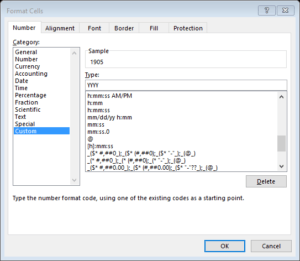Thanks for reading CFI’s guide to important Excel functions! By taking the time to learn and master these functions, you’ll significantly speed up your financial modeling. To learn more, check out these additional CFI resources:

• Excel Functions for Finance